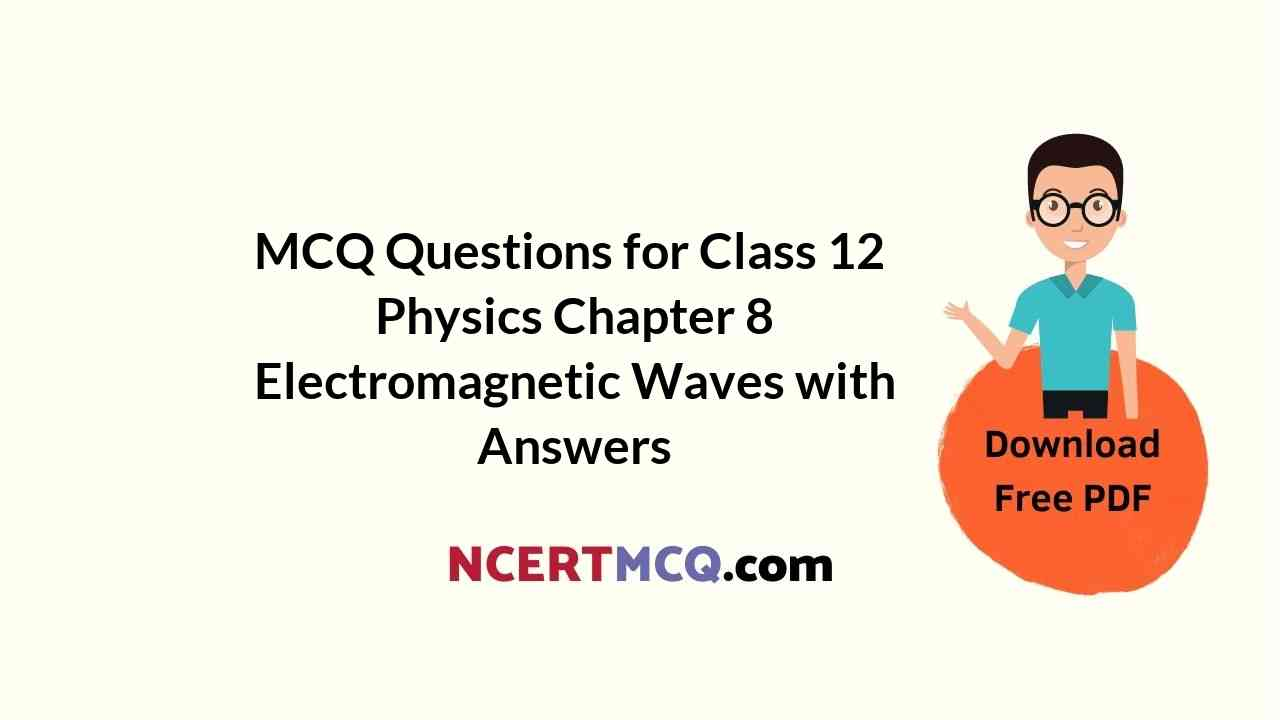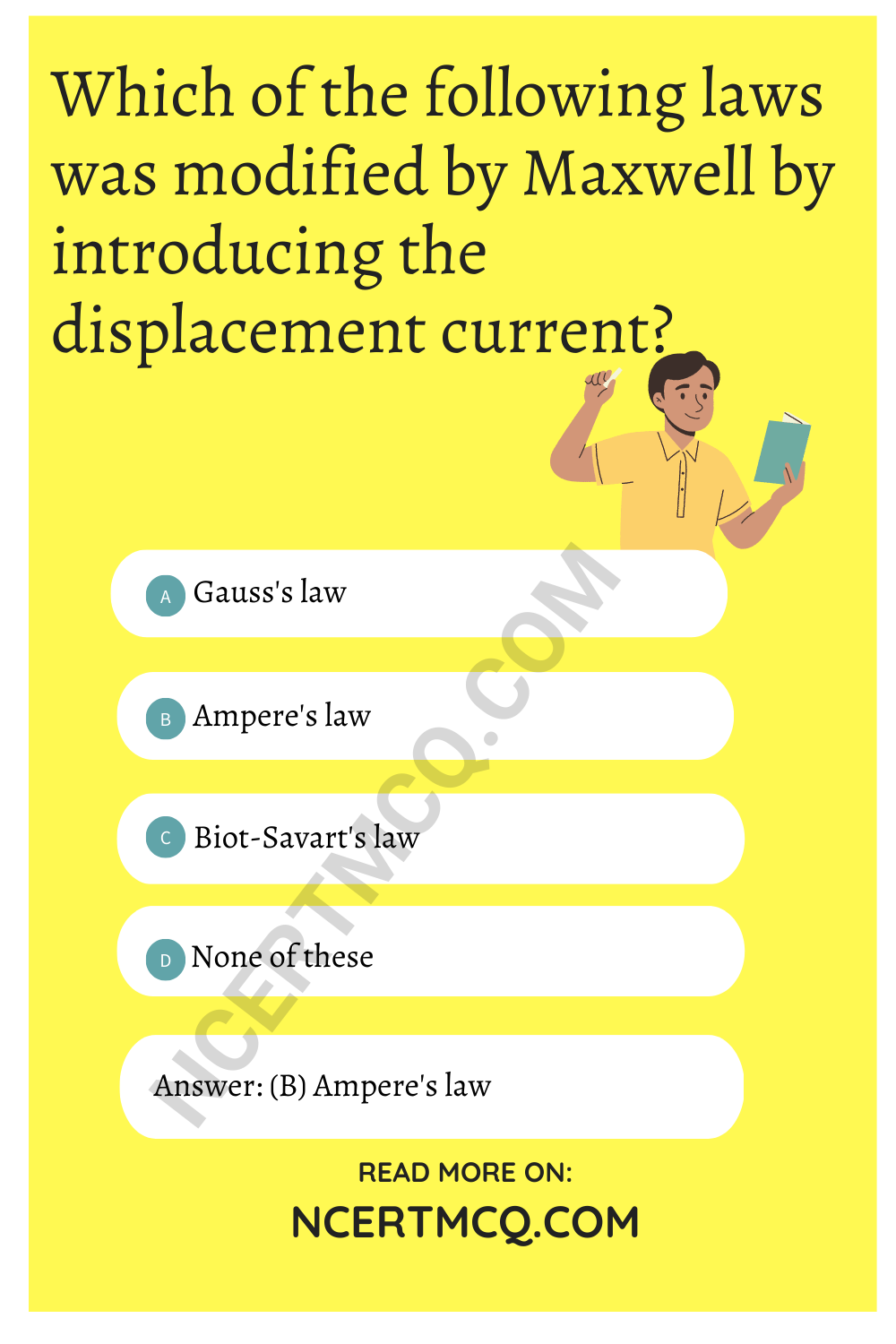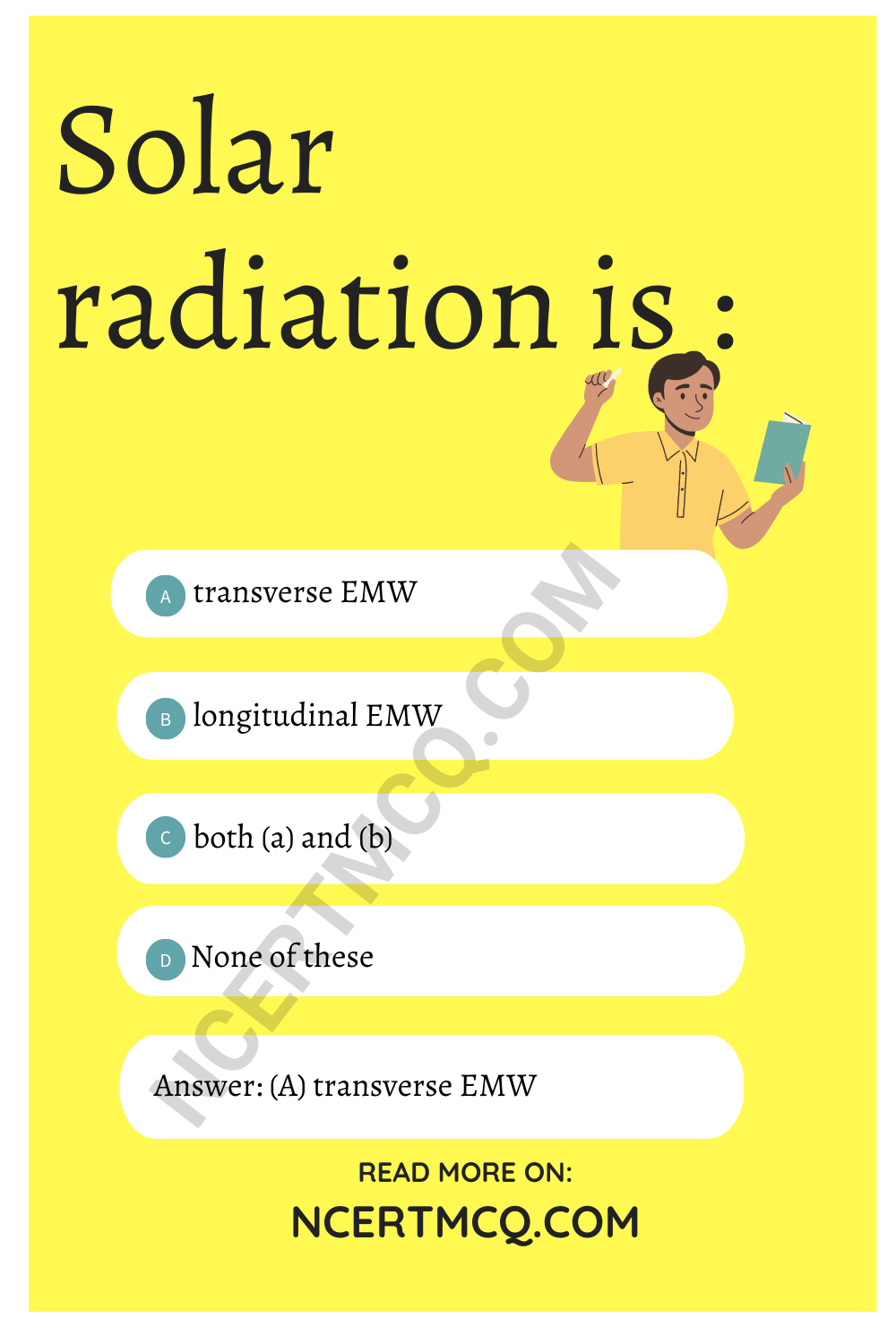Check the below NCERT MCQ Questions for Class 12 Physics Chapter 8 Electromagnetic Waves with Answers Pdf free download. MCQ Questions for Class 12 Physics with Answers were prepared based on the latest exam pattern. We have provided Electromagnetic Waves Class 12 Physics MCQs Questions with Answers to help students understand the concept very well.

## Electromagnetic Waves Class 12 MCQs Questions with Answers

MCQ On Electromagnetic Waves Class 12 Question 1.
The dimensional formula of $$\vec{E}$$ is:
(a)[MLT-2A-1]
(b) [MLT-3A-1]
(c) [MLT-2A-2]
(d) None of these

Electromagnetic Waves Class 12 MCQ Question 2.
The dimensional formula of is:
(a) [MLT-2A-1]
(b) [ML0T-2A-1]
(c) [ML0T-3A-1]
(d) None of these

Electromagnetic Waves MCQ Class 12 Question 3.
Which of the following has the dimensions of current?
(a) ε0 $$\vec{E}$$.$$\vec{dt}$$
(b) µ0 $$\vec{E}$$.$$\vec{dt}$$
(c) $$\frac{\vec{B}.\vec{dl}}{µ_0}$$
(d) $$\frac{\vec{B}.\vec{dl}}{ε_0}$$

Answer: (c) $$\frac{\vec{B}.\vec{dl}}{µ_0}$$

MCQ On Electromagnetic Waves Class 12 Question 4.
Which of the following has the dimensions of charge?
(a) $$\frac{ε_0\vec{E}}{\vec{ds}}$$
(b) µ0 $$\vec{E}$$.$$\vec{ds}$$
(c) $$\frac{ε_0}{µ_0}$$ $$\vec{E}$$.$$\vec{ds}$$
(d) $$\frac{µ_0}{ε_0}$$ $$\vec{E}$$.$$\vec{ds}$$

Answer: (a) $$\frac{ε_0\vec{E}}{\vec{ds}}$$

Class 12 Physics Chapter 8 MCQ Question 5.
Maxwell’s equation involving $$\frac{d}{dt}$$ $$\vec{B}$$ is obtained-from:
(a) Ampere’s law
(b) Gauss’s law
(d) Biot-Savart’s law

Electromagnetic Waves MCQ Pdf Class 12 Question 6.
The dimensions of $$\vec{E}$$, $$\vec{dl}$$ are identical to that of:
(a) potential
(b) charge
(c) current
(d) None of these

Electromagnetic Waves MCQ Questions Class 12 Question 7.
Which of the following laws was modified by Maxwell by introducing the displacement current?
(a) Gauss’s law
(b) Ampere’s law
(c) Biot-Savart’s law
(d).None of theseMCQ On Electromagnetic Wave With Answers Class 12 Question 8.
Choose the wave relevant to telecommunication :
(a) ultraviolet
(b) infrared
(c) microwave
(d) visible light

MCQs On Electromagnetic Waves Class 12 Question 9.
Electromagnetic waves have a speed of
(a) 3 × 105 kms-1
(b)3 × 106 kms-1
(c) 3 × 107 kms-1
(d) 3 × 108 kms-1

Answer: (a) 3 × 105 kms-1

Electromagnetic Waves Are Produced By MCQ Class 12 Question 10.
Which of the following EMW has highest wavelength?
(a) X-ray
(b) ultraviolet rays
(c) infrared rays
(d) microwaves

Electromagnetic Waves MCQs Class 12 Question 11.
Displacement current is due to:
(a) the flow of electrons
(b) the Varying electric field
(c) the ionization of atmosphere
(d) the flow of protons

Answer: (b) the Varying electric field

Electromagnetic Waves Objective Questions Class 12 Question 12.
Displacement current is always :
(a) equal to conduction current
(b) less than conduction current
(c) greater than conduction current
(d) the sum of current due to flow of positive and negative ions

Answer: (a) equal to conduction current

Electromagnetic Waves MCQs Class 12 Question 13.
EMW are produced by:
(a) charge in uniform motion only
(b) charge at rest only
(c) accelerated or decelerated charge only
(d) all of the above

Answer: (c) accelerated or decelerated charge only

Electromagnetic Wave MCQ Class 12 Question 14.
Who first demonstrated the existence of em waves?
(a) Hertz
(b) Maxwell
(c) Ampere

MCQ On Displacement Current Class 12 Question 15.
If $$\vec{B}$$ is the magnetic field vector and $$\vec{E}$$ is the electric field vector in an em wave, then which of the following relations is correct? Here c is the speed of fight:
(a) c = $$\frac{E}{B}$$
(b) c = BE
(c) c = $$\frac{B}{E}$$
(d) c = $$\frac{1}{EB}$$

Answer: (a) c = $$\frac{E}{B}$$

Question 16.
The dimensions of E/Bare same as that of:
(a) charge
(b) current
(c) velocity
(d) acceleration

Question 17.
Name the law which states that a varying electric field produces a magnetic field:
(a) Biot-Savart’s law
(c) Modified Ampere’s law
(d) None of these

Question 18.
The existence of EMW was experimentally established by :
(a) Sir S. C. Bose
(b) Maxwell
(c) Marconi
(d) Hertz

Answer: (a) Sir S. C. Bose

Question 19.
An EMW of v = 3 MHz passes from vacuum into dielectric medium with permittivity Er = 4, then:
(a) Wavelength is doubled and the frequency remains unchanged
(b) Wavelength is doubled and the frequency become half
(c) Wavelength is halved and the frequency remains unchanged
(d) Wavelength and frequency both remain unchanged

Answer: (c) Wavelength is halved and the frequency remains unchanged

Question 20.
Frequency of a wave is 6 × 1015. Hz. The wave is :
(b) Microwave
(c) X-ray
(d) Ultra violet

Question 21.
Choose the wrong statement?
(a) Electromagnetic waves are transverse
(b) Electromagnetic waves travel with the speed of light in free space
(c) Electromagnetic waves are produced by accelerating charges
(d) Electromagnetic waves travel with the same speed in all media

Answer: (d) Electromagnetic waves travel with the same speed in all media

Question 22.
If ue and um arc the electric and magnetic field densities in an electromagnetic wave, then (c is the speed of light):
(a) ue = um
(b) ue = c um
(c) ue = $$\frac{u_m}{c}$$
(d) None of these

Question 23.
(a) transverse EMW
(b) longitudinal EMW
(c) both (a) and (b)
(d) None of theseQuestion 24.
Ampere’s circuital law was modified by:
(a) Laplace
(b) Gauss
(c) Maxwell
(d) Hertz

Question 25.
Light Year is the unit of:
(a) distance
(b) time
(c) energy
(d) intensity of light